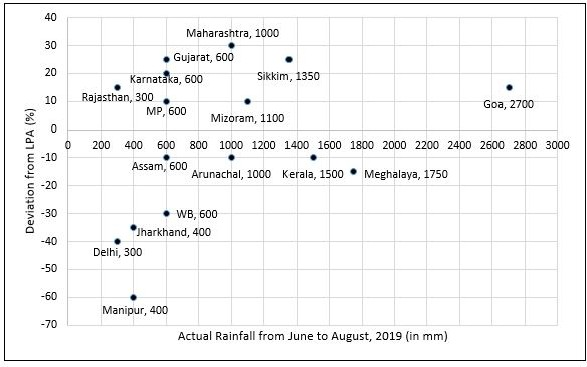### CAT 2019 – Slot 2- Logical Reasoning and Data Interpretation – To compare the rainfall data, India Meteorological Department (IMD) calculated the Long Period Average (LPA)

\$ 39
99
Monthly
• List Item #1
• List Item #2
Popular
##### STo compare the rainfall data, India Meteorological Department (IMD) calculated the Long Period Average (LPA) of rainfall during period June-August for each of the 16 states. The figure given below shows the actual rainfall (measured in mm) during June-August, 2019 and the percentage deviations from LPA of respective states in 2018. Each state along with its actual rainfall is presented in the figure..Q. 1:If a ‘Heavy Monsoon State’ is defined as a state with actual rainfall from June-August, 2019 of 900 mm or more, then approximately what percentage of ‘Heavy Monsoon States’ have a negative deviation from respective LPAs in 2019?
1. 42.86
2. 75.00
3. 14.29
4. 57.14

##### Q. 2: If a ‘Low Monsoon State’ is defined as a state with actual rainfall from June- August,2019 of 750 mm or less, then what is the median ‘deviation from LPA’ (as defined in the Y-axis of the figure) of ‘Low Monsoon States’?1. 10%2. -20%3. -10%4. -30%

Q. 3: What is the average rainfall of all states that have actual rainfall of 600 mm or less in 2019 and have a negative deviation from LPA?
1.  500 mm
2.  460 mm
3.  367 mm
4.  450 mm

Q. 4:The LPA of a state for a year is defined as the average rainfall in the preceding 10 years considering the period of June-August. For example, LPA in 2018 is the average rainfall during 2009-2018 and LPA in 2019 is the average rainfall during 2010-2019. It is also observed that the actual rainfall in Gujarat in 2019 is 20% more than the rainfall in 2009. The LPA of Gujarat in 2019 is closest to
1. 490 mm
2. 525 mm
3. 475 mm
4. 505 mm

1. Negative deviation as well as rainfall greater than 900 are Arunachal, Kerala, Meghalaya

Rainfall greater than 900 and positive deviations are Maharashtra, Mizoram, Sikkim, Goa

Hence % = 3/7 x 100 = 42.86

2. As we have a total of 9 states with low monsoon and 5 states with negative deviation among them, the state of assam will have the median value = -10%

3. As We can see that there are 5 states (Asam, WB, Jharkhand, Delhi and Manipur ) with rainfall less than 600 and negative deviation.

So Required Average of states with rainfall less than 600 and negative deviation is (600 + 600 + 400 + 300 + 400)/5 = 460

4. Gujrat in 2018 has rainfall of 600 anad deviation of 25% and 2019 is 20% more than 2009  2009 rainfall is 600/1.2 = 500mm.

As deviation is +25% so average 2009 – 2018 is 600/1.25 = 480

LPA 2019 = (480×10 – 500 + 600 )/10 = 490mm

Inspiring Education… Assuring Success!!

Ghatkopar | Borivali | Andheri | Online